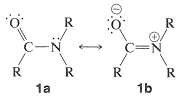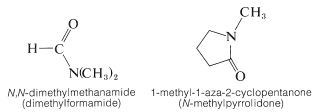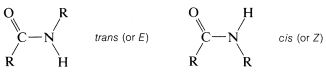# 24.1: Structural, Physical, and Spectral Characteristics of Amides

$$\newcommand{\vecs}{\overset { \rightharpoonup} {\mathbf{#1}} }$$ $$\newcommand{\vecd}{\overset{-\!-\!\rightharpoonup}{\vphantom{a}\smash {#1}}}$$$$\newcommand{\id}{\mathrm{id}}$$ $$\newcommand{\Span}{\mathrm{span}}$$ $$\newcommand{\kernel}{\mathrm{null}\,}$$ $$\newcommand{\range}{\mathrm{range}\,}$$ $$\newcommand{\RealPart}{\mathrm{Re}}$$ $$\newcommand{\ImaginaryPart}{\mathrm{Im}}$$ $$\newcommand{\Argument}{\mathrm{Arg}}$$ $$\newcommand{\norm}{\| #1 \|}$$ $$\newcommand{\inner}{\langle #1, #2 \rangle}$$ $$\newcommand{\Span}{\mathrm{span}}$$ $$\newcommand{\id}{\mathrm{id}}$$ $$\newcommand{\Span}{\mathrm{span}}$$ $$\newcommand{\kernel}{\mathrm{null}\,}$$ $$\newcommand{\range}{\mathrm{range}\,}$$ $$\newcommand{\RealPart}{\mathrm{Re}}$$ $$\newcommand{\ImaginaryPart}{\mathrm{Im}}$$ $$\newcommand{\Argument}{\mathrm{Arg}}$$ $$\newcommand{\norm}{\| #1 \|}$$ $$\newcommand{\inner}{\langle #1, #2 \rangle}$$ $$\newcommand{\Span}{\mathrm{span}}$$

## Molecular Structure

The structural parameters of the amide group have been determined carefully and the following diagram gives a reasonable idea of the molecular dimensions:An important feature of the group is that it is planar - the carbon, oxygen, nitrogen, and the first atom of each of the $$\ce{R}$$ groups on carbon and nitrogen lie in the same plane. The $$\ce{C-N}$$ bond distance of $$1.34 \: \text{Å}$$ is intermediate between the typical single bond $$\ce{C-N}$$ distance of $$1.47 \: \text{Å}$$ and the double bond $$\ce{C=N}$$ distance of $$1.24 \: \text{Å}$$. This and other evidence indicates that the amide group is a hybrid structure of the valence-bond forms $$1a$$ and $$1b$$, with stabilization energy of about $$18 \: \text{kcal mol}^{-1}$$:Coplanarity is required if the dipolar structure $$1b$$ is to be significant. An appreciable dipole moment may be expected of amides and, in fact, simple amides have dipole moments in the range 3.7-3.8 debye. (For reference, the carbonyl group has a moment of about 2.7 debye, Section 16-1B.)

As a consequence of the polarity of the amide group, the lower-molecular-weight amides are relatively high-melting and water-soluble, as compared to esters, amines, alcohols, and the like. The few that are liquids, such as $$\ce{N}$$,$$\ce{N}$$-dimethylmethanamide and 1-methyl-1-aza-2-cyclopentanone, have excellent solvent properties for both polar and nonpolar substances. Therefore they are good solvents for displacement reactions of the $$S_\text{N}$$ type (Table 8-5).Another very important consequence of amide structure is the extensive molecular association of amides through hydrogen bonding. The relatively negative oxygens act as the hydrogen acceptors while $$\ce{N-H}$$ hydrogens serve as the hydrogen donors:## Nomenclature

The naming of amides is summarized in Section 7-7D. The points to remember are that they generally are named either as (i) alkanamides, in which the prefix alkan(e) is determined by the longest carbon chain that includes the carbonyl group ($$\ce{HCONH_2}$$ is methanamide), or as (ii) substituted carboxamides, $$\ce{RCONH_2}$$, in which the name is completed by identifying the $$\ce{R}$$ substituent:The degree of substitution on the amide nitrogen determines whether the amide is primary, $$\ce{RCONH_2}$$, secondary, $$\ce{RCONHR}$$, or tertiary, $$\ce{RCONR_2}$$. When the amide is secondary or tertiary, the symbol $$\ce{N}$$ (for nitrogen) must precede the name of each different group attached to nitrogen:## Infrared Spectra

Considerable information is available on the infrared spectra of amides. By way of example, the spectra of three typical amides with different degrees of substitution on nitrogen are shown in Figure 24-1.Figure 24-1: Infrared spectra of propanamide, $$\ce{N}$$-phenylethanamide, and $$\ce{N}$$,$$\ce{N}$$-dimethylmethanamide in chloroform solution. Notice the appearance of both free $$\ce{NH}$$ bands (sharp, $$3300$$-$$3500 \: \text{cm}^{-1}$$) and hydrogen-bonded $$\ce{NH}$$ bands (broad, $$3100$$-$$3300 \: \text{cm}^{-1}$$) for primary and secondary amides.

A strong carbonyl absorption is evident in the spectra of all amides, although the frequency of absorption varies somewhat with the structure of the amide. Thus primary amides generally absorb near $$1680 \: \text{cm}^{-1}$$, whereas secondary and tertiary amides absorb at slightly lower frequencies. The $$\ce{N-H}$$ stretching frequencies of amides are closely similar to those of amines and show shifts of $$100 \: \text{cm}^{-1}$$ to $$200 \: \text{cm}^{-1}$$ to lower frequencies as the result of hydrogen bonding. Primary amides have two $$\ce{N-H}$$ bands of medium intensity near $$3500 \: \text{cm}^{-1}$$ and $$3400 \: \text{cm}^{-1}$$, whereas secondary amides, to a first approximation, have only one $$\ce{N-H}$$ band near $$3440 \: \text{cm}^{-1}$$. However, a closer look reveals that the number, position, and intensity of the $$\ce{N-H}$$ bands of monosubstituted amides depend on the conformation of the amide, which can be either cis or trans:Normally, the trans configuration is more stable than the cis conformation for primary amides. However, for cyclic amides (lactams), in which the ring size is small, the configuration is exclusively cis:## NMR Spectra

The proton NMR resonances of the $$\ce{N-H}$$ protons of amides are different from any we have discussed so far. Generally, these will appear at room temperature as a broad singlet absorption, which may turn into a broad triplet at higher temperatures. A typical example is propanamide (Figure 24-2).Figure 24-2: Proton NMR spectrum of propanamide, $$\ce{CH_3CH_2CONH_2}$$, in chloroform solution (solvent not shown) at $$60 \: \text{MHz}$$ relative to TMS at $$0.0 \: \text{ppm}$$.

The broad $$\ce{N-H}$$ proton resonance is due to the special nuclear properties of $$\ce{^{14}N}$$, the predominant natural isotope of nitrogen. This is established beyond question by observation of the proton spectrum of an amide in which the $$\ce{^{14}N}$$ is replaced by the $$\ce{^{15}N}$$ isotope to give $$\ce{RCO} \ce{^{15}N} \ce{H_2}$$. In this case the proton lines are sharp. The details of the phenomena that lead to the broad resonances of the $$\ce{N-H}$$ protons in amides are discussed elsewhere;$$^1$$ for our purposes it should suffice to note that the $$\ce{^{14}N}$$ nucleus has much shorter lifetimes for its magnetic states than do protons, and the broad lines result from uncertainties in the lifetimes of the states associated with $$\ce{^{14}N} \ce{-H}$$ spin-spin coupling (Section 27-1). One should be prepared for absorptions of this character in amides and some other substances with $$\ce{N-H}$$ bonds that are not involved in rapid intermolecular proton exchanges, which, when sufficiently rapid, have the effect of averaging the magnetic effects of the $$\ce{^{14}N}$$ atoms to zero:

$\ce{R'NH_2} + \ce{R} \overset{\oplus}{\ce{N}} \ce{H_3} \rightleftharpoons \ce{R'} \overset{\oplus}{\ce{N}} \ce{H_3} + \ce{RNH_2}$

or

$\ce{R'NH_2} + \ce{R} \overset{\ominus}{\ce{N}} \ce{H} \rightleftharpoons \ce{R'} \overset{\ominus}{\ce{N}} \ce{H} + \ce{RNH_2}$

The situation here is analogous to that discussed for the splitting of the resonances of $$\ce{O-H}$$ protons of alcohols by protons on the $$\alpha$$ carbons (see Section 9-10I).

The NMR spectra of amides are revealing as to the structure of the amide group. For example, the spectrum of $$\ce{N}$$,$$\ce{N}$$-dimethylmethanamide shows two three-proton single resonances at $$2.78 \: \text{ppm}$$ and $$2.95 \: \text{ppm}$$, which means that at ordinary temperatures the two methyl groups on nitrogen are not in the same molecular environment:This is a consequence of the double-bond character of the $$\ce{C-N}$$ bond expected from valence-bond structures $$1a$$ and $$1b$$, which leads to restricted rotation about this linkage. One of the methyl groups (A) has a different stereochemical relationship to the carbonyl group than the other methyl group (B). Groups A and B therefore will have different chemical shifts, provided that rotation about the $$\ce{C-N}$$ bond is slow. However, at $$150^\text{o}$$ the two three-proton lines are found to coalesce to a single six-proton line, which means that at this temperature bond rotation is rapid enough to make the methyl groups essentially indistinguishable (see Section 9-10C):Most amides do not rotate freely about the $$\ce{C-N}$$ bond. The barrier to this kind of rotation is about $$19 \: \text{kcal mol}^{-1}$$, which is high enough for the nonequivalence of groups on nitrogen to be observable by spectral techniques, but not quite high enough to allow for actual physical separation of stable $$E,Z$$ configurational isomers.

$$^1$$J. D. Roberts, Nuclear Magnetic Resonance, Applications to Organic Chemistry, McGraw-Hill Book Co., New York, 1959, Chapter 5. Also see the NMR references at the end of Chapter 9 in this book.